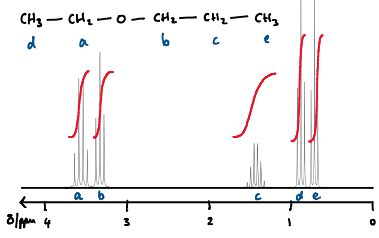# Predict the integration for each proton in the 1H NMR spectrum for...

## Question:

Predict the integration for each proton in the {eq}1H NMR {/eq} spectrum for {eq}CH_{3}{/eq}{eq}-CH_{2}{/eq}{eq}-O-{/eq}{eq}CH_{2}{/eq}{eq}-CH_{2}{/eq}{eq}-CH_{3}{/eq}. Please provide rule to predict integrations and explain how you find them.

## Integration Traces on 1H NMR Spectra:

Proton (1H) NMR spectra consist of a series of peaks at different chemical shifts, corresponding to different chemical environments of the protons in a molecule. The total area under each multiplet in the spectrum is called the integration. The value of the integration is proportional to the number of protons in the chemical environment giving rise to the signal observed. The integration values are usually shown on a spectrum with an 'integrator trace,' computed by software. This is a graph-like depiction; the length of the line drawn across the peak corresponds to the relative integration value. This depiction is useful because all the lengths in the spectrum can be compared to give the ratio of the number of protons in each environment.

The origin of the relationship of peak area being related to the number of protons is due to quantity on the vertical axis in the spectrum. This is actually intensity (not usually labelled on a typical NMR spectrum). The more protons in an environment, the more intense the signal picked up by the machine will be, and so the greater area of the peak. Area is a more accurate value to use than peak height.

Firstly, we need to work out what the spectrum will look like for the molecule {eq}CH_3CH_2OCH_2CH_2CH_3{/eq}. To develop it, we need to do the following:

1. Find the number of chemical environments for the protons (hydrogens). Here, there are 5.

2. Determine the multiplicities for each environment. This is done by counting the number of protons the environment is coupled to (count the number of protons (hydrogens) within three bonds. Next apply the n+1 rule, e.g. if there are 2 protons within three bonds, then the peak structure will be a triplet, since by n+1, 2+1=3 peaks. Going from left to right in the formula of the molecule the multiplicities are triplet, quartet, triplet, sextet, triplet.

3. Place these peaks at the appropriate position on the chemical shift (horizontal) axis of the spectrum. Don't worry too much about exactly what value in ppm these will be at, because this is rather advanced to determine. However, the order should be easy to predict since it depends on how deshielded the protons in each environment are. For this case, the oxygen atom is electronegative so will attract electrons towards it. Therefore, the {eq}CH_2{/eq} groups next to the oxygen atom will have the highest chemical shift values. The correct order is shown on the spectrum below. The peaks corresponding to each environment are labelled a, b, c, d, e. From left to right in the formula of the molecule, {eq}CH_3CH_2OCH_2CH_2CH_3{/eq}, the peak for each set of hydrogens is d, a, b, c, e.

Now that we have the spectrum, we can think about what the integrator trace will look like. The integration for each multipet in the spectrum is given by the number of hydrogens in the environment. Therefore, d and e will have an integration value of 3, and a, b, and c will have an integration value of 2. This is usually described as a ratio and the spectrum would be described as having an integration ratio of 2:2:2:3:3. The integrator trace is the red line on the spectrum below. The ratio of heights is proportional to the ratio of protons in each environment.